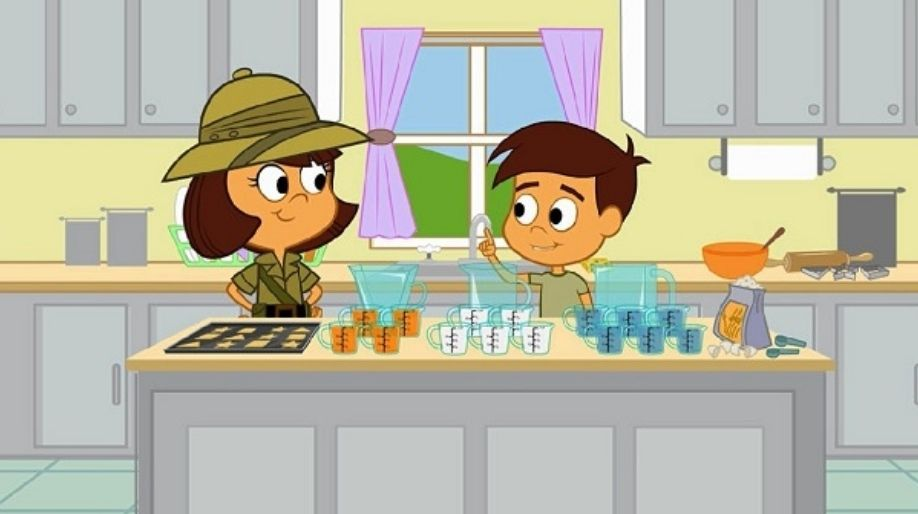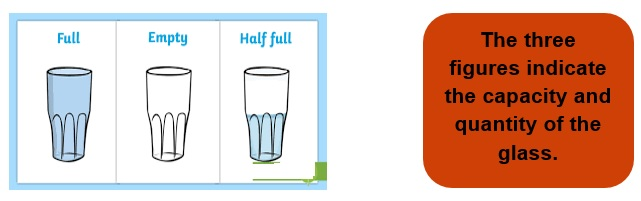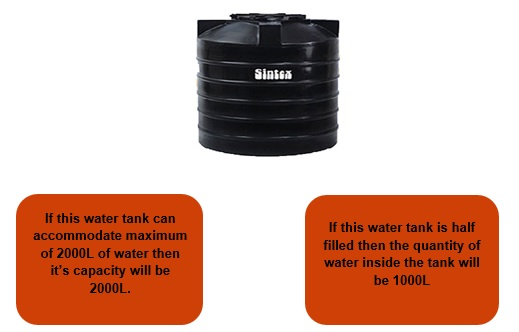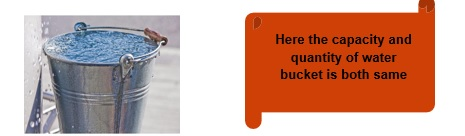Register for Maths, Science, English, Reasoning Olympiad Exams Register here | Book Free Demo for Live Olympiad Classes here | Check Olympiad Exam Dates here | Buy Practice Papers for IMO, IOM, HEO, IOEL etc here | Login here to participate in all India free mock test on every Saturday

# Capacity and QuantityHave you ever thought while filling a water bottle how much water it can accommodate and when the shape of bottle is changed the quantity of water also changes. Come along we’ll find the answers to these and some other interesting questions.• Capacity of any solid or any object can be defined as the maximum amount of liquid or any other pourable substance that can be stored inside the solid without any leakage from the solid.
• It simply means that just like a glass can contain only a limited quantity of water similarly every other object has a maximum limit to which they can store water.
• The amount of liquid or any other pourable substance stored in the object or solid is known as quantity of that liquid.
• Capacity and Quantity have the same standard unit Liter (L) or Milliliter (ml).

Let’s have an example of water tanks stored at our house to understand these two terms clearly.Units and Its Conversions

The capacity and quantity both of the same units as they are the measurements of a pourable substance like amount of liquid inside a solid object.

So they are measure either in Liters (L) or Milliliters (ml)

• The standard unit is L.

• For measuring small quantities we use the unit milliliter and for measuring large quantities we use the unit Liter.

• For example for measuring the amount of milk in a glass we will use the unit ml but for measuring the amount of water in a bucket we will use the unit L.

Formula:

 1 Liter = 1000 ml 1 ml = 1/1000 L

Example 1: Convert 12 L to ml.

Solution: As we know that

1 L = 1000 ml

Therefore 12 L = (12 x 1000) ml

= 12000 ml.

Example 2: A bucket has a capacity of 20 L. It is half filled with water. Find the quantity of water in the bucket and express it in ml.

Solution: As we know that 1 L = 1000 ml.

Therefore 20 L = (20 x 1000) = 20,000 ml.

Hence the capacity of bucket is 20,000 ml.

Since the bucket is half filled which means

Quantity of water present will be = 20,000 ÷ 2 = 10,000 ml.

Example 3: A water bottle has a capacity of 20,000 ml. It is filled to 1/4th of its capacity. Find the quantity of water present in the bottle.

Solution: The capacity of water bottle is 20,000ml

And 1 ml = 1/1000 L

Therefore, 20,000 ml = 20,000 x 1/1000 = 20L.

Hence the capacity of water bottle is 20L.

Since the water bottle is filled with 1/4th of the capacity of water bottle which means

(1/4 x 20) L = 5L

Hence the quantity of water in the bottle is 5L.

• If any object is fully filled then in this case capacity is equal to its quantity.
• Capacity is always about an object or solid whereas quantity is always measured for the pourable substance present inside the object like liquid or gas present inside the object.For Example we always say that the capacity of a water bucket is 20 L or 30 L and the quantity of water in it is 12 L or 18 L.

• So capacity is related to object or containers whereas quantity is related to the pourable substances like gases or liquids.
• Quantity will be always less than or equal to the value of Capacity.

Example 5: If a bucket has 24 L of water in it and another bucket has 12 L of water. Find the total quantity of water present inside the buckets.

Solution: The total quantity of water will be =

Quantity of water in 1st bucket + Quantity of water in 2nd bucket

= (24 + 12) L = 36 L.

Practice Questions

Q1) Convert the following quantities to Litre.

1. 123 ml
2. 5600 ml
3. 4000 ml

Q2) Convert the following to mililitres.

1. 25 L
2. 2 L
3. 32 L
4. 256 L

Q3) State True or False:

1. 1 L = 1000 ml
2. 1 ml = 1/1000 L
3. Capacity is less than or equal to Quantity.
4. Water in a can should me measure in Litres.

Q4) How many 200 ml of bottles can fill a 2 Litre drum?

Recap

• Capacity and Quantity are measurements values.
• They are always measured in L or ml.
• 1 L = 1000 ml.
• Capacity is always greater than or equal to the value of Quantity.
• For smaller measurements we use the unit ml and for larger we use the unit L.
• The standard unit is Liters or L.

## Quiz for Capacity and Quantity

 Q.1 Sanket consumed 15 L of water in 2 days and 27 litres of water in the rest of the days of the week. How much water was consumed in a week by him? a) 41 L b) 40 L c) 42 L d) 43 L
 Q.2 The standard unit of capacity is: a) millilitre b) litre c) kilograms d) grams
 Q.3 1 litre =_______________ millilitre. a) 1 ml b) 1000 ml c) 100 ml d) 10 ml
 Q.4 The quantity of liquid that a vessel can contain or hold is its ________________. a) capacity b) liquid c) litre d) millilitre
 Q.5 The unit of measurement for syrup is _____________. a) ml b) l c) g d) mg
 Q.6 Harry had 3 buckets of different capacity which could hold 9250 ML, 12420 MLand 30100 ML of water. How much water could be stored in all? a) 51 L 771 ML b) 51 L 769 ML c) 51 L 770 ML d) 50 L 770 ML
 Q.7 The unit of measurement of capacity of a container is _____ and ______. a) litre, millilitre b) kilogram, gram c) metre, centimetre d) none of the above
 Q.8 Neha purchased 500 mL bottle of Pepsi, 300 mL can of Frooti and 2 L of Limca. What quantity of cold drinks did Neha purchases altogether? a) 2.5 L b) 2.6 L c) 2.7 L d) 2.8 L
 Q.9 Convert the following into millilitres: 17 litres a) 1.7 ml b) 17 ml c) 1700 ml d) 17000 ml
 Q.10 Convert the following into millilitres: 8 litres a) 800 ml b) 8000 ml c) 80 ml d) 8 ml